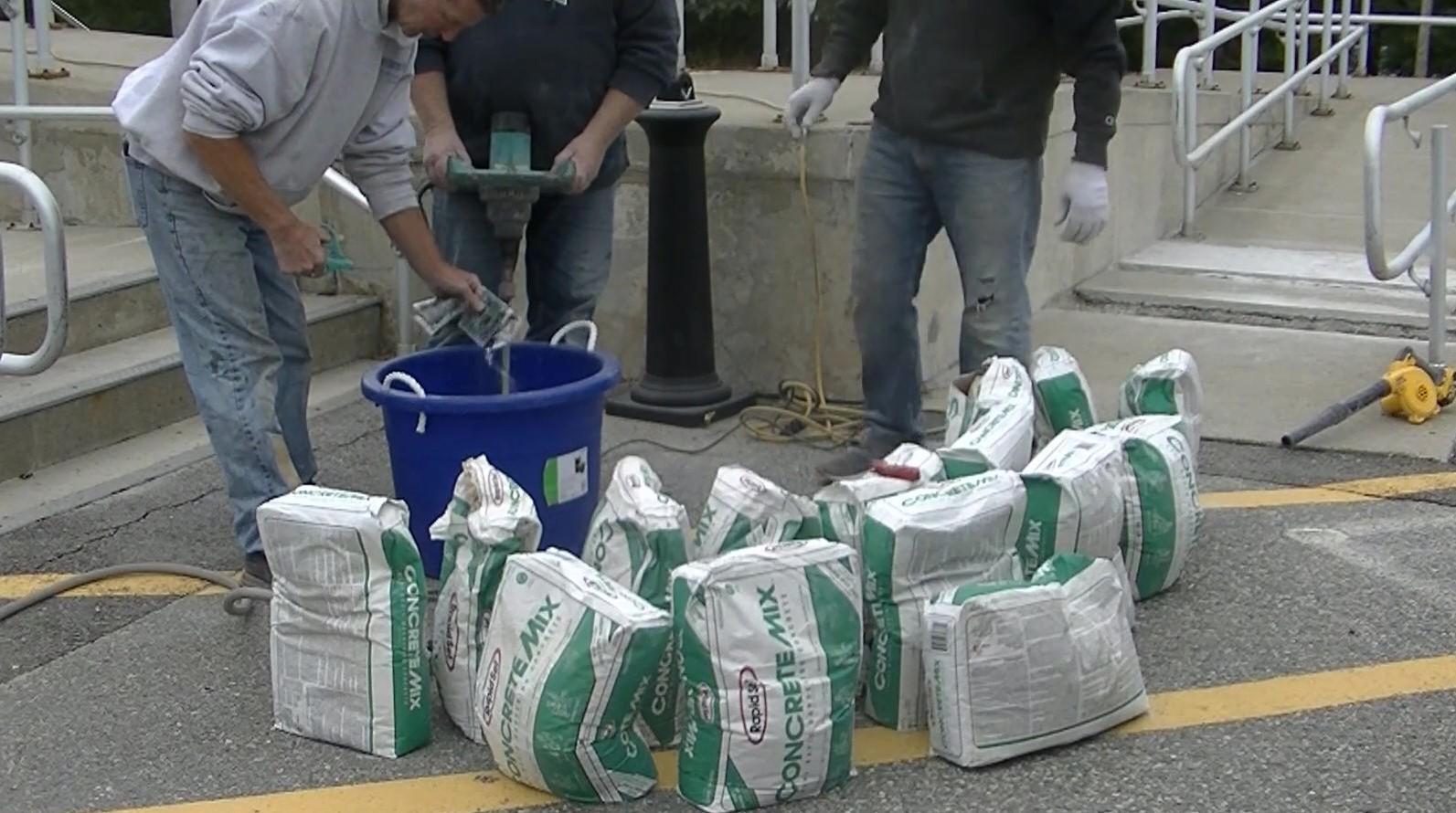# concrete bag mix Calculator## calculate how many bags of concrete you need

Scroll down to see the calculator!

Here's a free concrete bag mix calculator to help you figure the amount of cement or concrete you need to do your project.

Most people call concrete "cement" when in fact cement is just one ingredient along with sand, aggregate and water that is used to make concrete.

If you're mixing the concrete by hand and you need to calculate the amount of Pre-Mixed concrete bags needed to do your project, this calculator will also figure the amount of bags you need.

Just enter your thickness, length, and width, then click on "calculate" and the calculator will show you how many 40 lb, 60 lb, and 80 lb bags you need to do your slab.

You can use these totals to figure how many bags of Sakrete or Quikrete you need for the size job you have.

When ordering concrete from a redi-mix supplier, the concrete comes in cubic yards.  If you tell the Dispatcher your dimensions and thickness, he can figure the concrete for you as well.

It's important to accurately calculate the amount of concrete for a project because it's very expensive to buy from a redi-mix company.  You don't want to order to much, and you don't want to run short.

There is a formula to figure the amount of concrete for a slab, floor, or patio.  The formula is in feet and is L x W x H (/27). This formula will give you the estimated cubic yardage needed to do your project.

For example if you have a concrete slab 24' L x 24' W x 6" thick, the formula would read like this; 24 x 24 = 576 x .5 = 288 divided by 27 = 10.67 cubic yards.  (make sure you change the inches thick to feet, 6 inches thick = .5 feet)

It's always a good idea to check your figures once in a while using a pencil and paper, typing in a wrong number on the calculator could lead to you over or under estimating the amount of concrete you need.

Return from Cement Calculator to Concrete Calculator

One more way to share everything-about-concrete.com